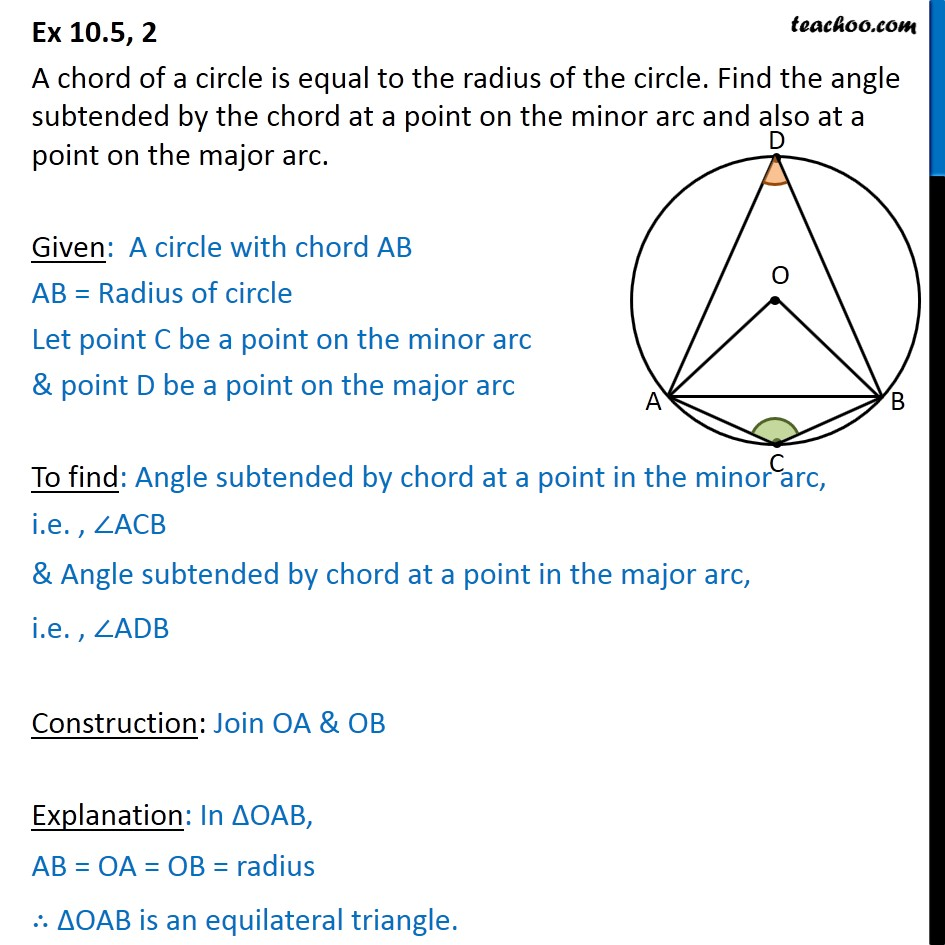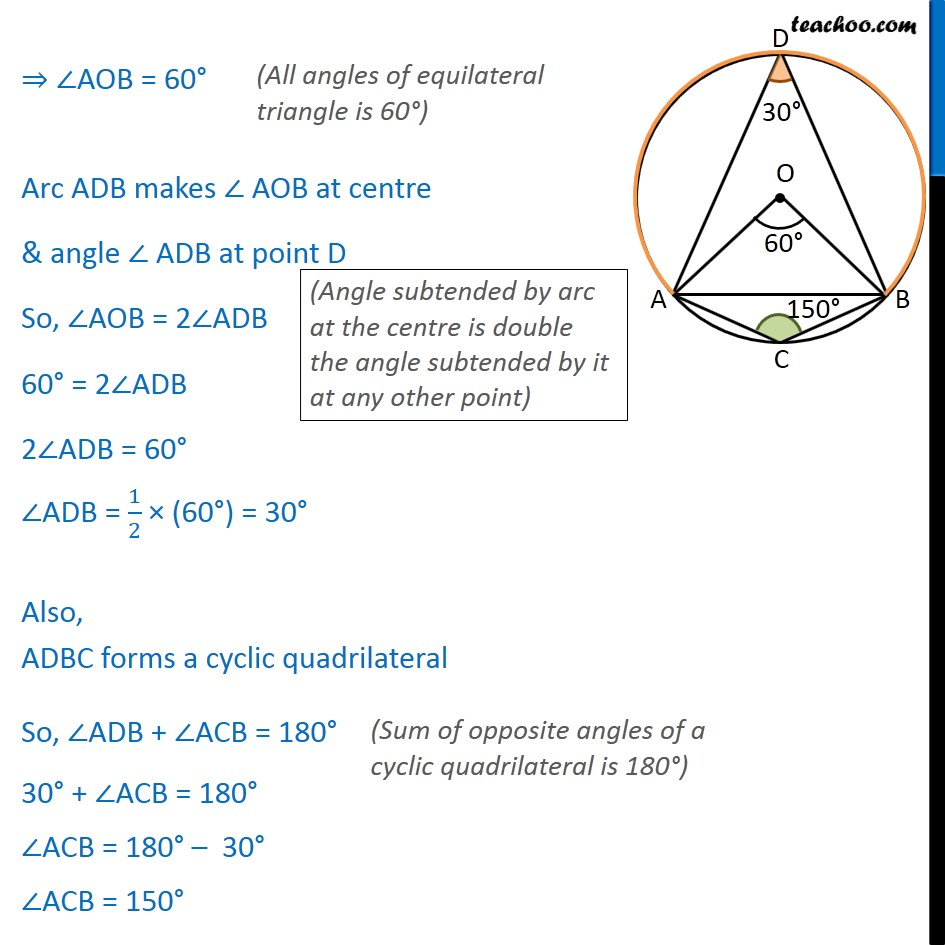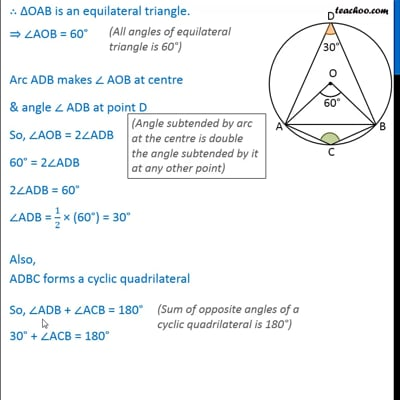Ex 10.5

Chapter 10 Class 9 Circles
Serial order wiseThis video is only available for Teachoo black users

Solve all your doubts with Teachoo Black (new monthly pack available now!)

### Transcript

Ex 10.5, 2 A chord of a circle is equal to the radius of the circle. Find the angle subtended by the chord at a point on the minor arc and also at a point on the major arc. Given: A circle with chord AB AB = Radius of circle Let point C be a point on the minor arc & point D be a point on the major arc To find: Angle subtended by chord at a point in the minor arc, i.e. , ∠ACB & Angle subtended by chord at a point in the major arc, i.e. , ∠ADB Construction: Join OA & OB Explanation: In ΔOAB, AB = OA = OB = radius ∴ ΔOAB is an equilateral triangle. ⇒ ∠AOB = 60° Arc ADB makes ∠ AOB at centre & angle ∠ ADB at point D So, ∠AOB = 2∠ADB 60° = 2∠ADB 2∠ADB = 60° ∠ADB = 1/2 × (60°) = 30° Also, ADBC forms a cyclic quadrilateral So, ∠ADB + ∠ACB = 180° 30° + ∠ACB = 180° ∠ACB = 180° – 30° ∠ACB = 150°